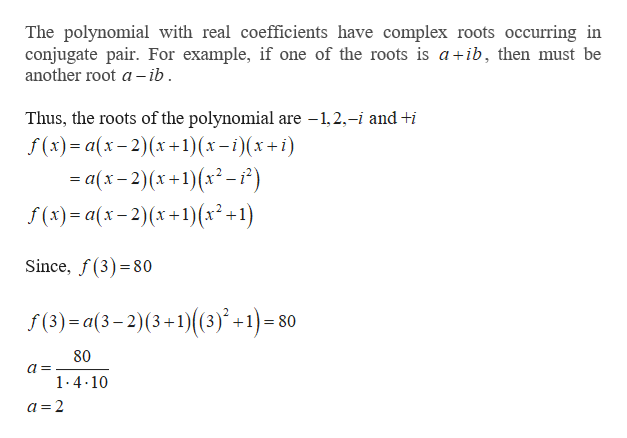# Find a polynomial function f(x) having -1,2, and + i as zeros and f(3)=80

Question
15 views

Find a polynomial function f(x) having -1,2, and + i as zeros and f(3)=80

check_circle

Step 1

Given information:

Step 2

Find the polynomial func...help_outlineImage TranscriptioncloseThe polynomial with real coefficients have complex roots occurring in conjugate pair. For example, if one of the roots is a+ib, then must be another root a -ib Thus, the roots of the polynomial are -1,2,-i and +i f(x) a(x-2)x1)(x-i)(x+ i) = a(x-2)(x+1)(x2-2) f(x) a(x-2)(x+1)(x2+1) Since, f(3)80 f (3) a(3-2)(3+1)(3) +1)=80 80 1-4.10 a=2 fullscreen

### Want to see the full answer?

See Solution

#### Want to see this answer and more?

Solutions are written by subject experts who are available 24/7. Questions are typically answered within 1 hour.*

See Solution
*Response times may vary by subject and question.
Tagged in

### Polynomials